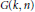Hostname: page-component-546b4f848f-gfk6d Total loading time: 0 Render date: 2023-06-03T10:47:52.337Z Has data issue: false Feature Flags: { "useRatesEcommerce": true } hasContentIssue false

# Schubert Calculus on a Grassmann Algebra

Published online by Cambridge University Press:  20 November 2018

## Abstract

HTML view is not available for this content. However, as you have access to this content, a full PDF is available via the ‘Save PDF’ action button.

The (classical, small quantum, equivariant) cohomology ring of the grassmannian$G\left( k,\,n \right)$ is generated by certain derivations operating on an exterior algebra of a free module of rank$n$ (Schubert calculus on a Grassmann algebra). Our main result gives, in a unified way, a presentation of all such cohomology rings in terms of generators and relations. Using results of Laksov and Thorup, it also provides a presentation of the universal factorization algebra of a monic polynomial of degree$n$ into the product of two monic polynomials, one of degree$k$.

## Keywords

Type
Research Article
Information
Canadian Mathematical Bulletin , 01 June 2009 , pp. 200 - 212

## References

 Bertram, A., Quantum Schubert calculus. Adv. Math. 128(1997), no. 2, 289305.CrossRefGoogle Scholar
 Fulton, W., Intersection Theory. Ergebnisse der Mathematik und ihrer Grenzgebiete 3, Springer-Verlag, Berlin, 1984.CrossRefGoogle Scholar
 Fulton, W., Equivariant Intersection Theory. The Eilenberg Lectures at Columbia University, 2007. Notes by D. Anderson available at http://www.math.lsa.umich.edu/_dandersn/eilenberg.Google Scholar
 Gatto, L., Schubert calculus via Hasse–Schmidt derivations. Asian J. Math. 9(2005), no. 3, 315321.CrossRefGoogle Scholar
 Gatto, L., Schubert calculus: an algebraic introduction. IMPA Mathematical Publications, Rio de Janeiro, 2005.Google Scholar
 Gatto, L. and Santiago, T., Equivariant Schubert Calculus. http://calvino.polito.it/_gatto/public/lavori/preprints/equivariant.pdf. Google Scholar
 Knutson, A. and Tao, T. Puzzles and (equivariant) cohomology of Grassmannians. Duke Math. J. 119(2003), no. 2, 221260.Google Scholar
 Laksov, D., The formalism of equivariant Schubert calculus. Adv. Math 217(2008), 18691888.CrossRefGoogle Scholar
 Laksov, D. and Thorup, A., A determinantal formula for the exterior powers of the polynomial ring. Indiana Univ. Math. J. 56(2007), 825846.CrossRefGoogle Scholar
 Laksov, D. and Thorup, A., Schubert calculus on Grassmannians and exterior products. To appear in Indiana Univ. Math. J.Google Scholar
 Mihalcea, L. C., Giambelli formulae for the equivariant quantum cohomology of the Grassmannian. Trans. Am. Math. Soc. 360(2008), no. 5, 22852301.CrossRefGoogle Scholar
 Santiago, T., Schubert calculus on a Grassmann algebra. Ph.D. Thesis, Politecnico di Torino, 2006.Google Scholar
 Siebert, B. and Tian, G., On quantum cohomology rings of Fano manifolds and a formula of Vafa and Intrilligator. Asian J. Math. 1(1997), no. 4, 679695.CrossRefGoogle Scholar
 Witten, E., The Verlinde algebra and the cohomology of the Grassmannian. In: Geometry, topology and physics, Conf. Proc. Lecture Notes Geom. Topology IV, International Press, Cambridge, MA, 1995, pp. 357422.Google Scholar